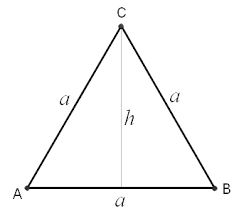# C Program Area Of Equilateral Triangle | C Programs

C Program to find the area of an equilateral triangle – In this particular article, we will detail in on the various methods to calculate the area of an equilateral triangle.

The several methods used to do so are as follows:

• Using Standard Method
• Using Function
• Using Pointers
• Using Macros

In this blog piece, we have added suitable examples and sample programs for your better understanding. The compiler has been added as well so that you can execute the codes.

As you all know, an equilateral triangle is a triangle whose all three sides are of equal length.

Because of this very fact, all the angles of an equilateral triangle are equal as well, i.e. 60 degrees.

This is how an equilateral triangle looks like:As you can see, this is an equilateral triangle with a side length of “a” units and a height of “h” units.

The altitude of an equilateral triangle cuts the opposite side at right angles. So, by using the Pythagoras theorem, we can calculate the height of an equilateral triangle to be sqrt(3/4)a.

Thus, the area of an equilateral triangle = 1/2 * sqrt (3/4)a * a

Area = (sqrt 3)/4 * a^2

Hence, the various methods to calculate the area of an equilateral triangle in C programming are as follows:

## Using Standard Method

1)For calculating the area of an equilateral triangle, we need the only side of the triangle.

2)The value of side will store into the variable “a”, and “a” value will be substituted into the formula, then we get the area value.

output:

### Using Functions

1)We are using the function area(float a) to calculate the area of an equilateral triangle, where the return type is a float.

2)area(a) is calling the function which is having float datatype argument, here area(float a) having float datatype argument “a”

3)area(float a) calculate the area of an equilateral triangle and return the area value, that value will be assigned to the variable “Ar”.

output:

#### Using Pointers

1)We are calling the function as area(&a,&Ar), here we are passing the references/addresses of variables a,Ar.

2)The function area(float *a, float *Ar) will calculate the area of an equilateral triangle, which is having pointers as arguments.

3)The calculated value will store into the pointer variable “Ar”

output:
##### Using Macros

1)Line 3 represents area(a) is symbolic name to the expression (sqrt(3)/4)*a*a.

2)area(a)replaced with that expression given at #define.

output:
x

## C Program To Print Number Of Days In A Month | Java Tutoring

C program to input the month number and print the number of days in that ...